# 用导数来求极大值和极小值（但不是所有平坦的地方都是极大值或极小值，也可以有个鞍点)

（你也许想先去阅读关于 导数 的内容。）

### 例子：向上抛一个球。在球离开手 t 秒后，它的高度是：

h = 3 + 14t − 5t2

### 那么球最高的高度是多少？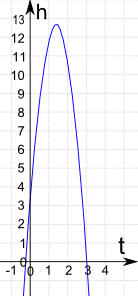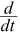h = 0 + 14 − 5(2t)
= 14 − 10t

（对于这个例子你可在下面看到怎样求这个导数。）

14 − 10t = 0
10t = 14
t = 14 / 10 = 1.4

h = 3 + 14×1.4 − 5×1.42
h = 3 + 19.6 − 9.8 = 12.8

### 简略重温导数

h = 3 + 14t − 5t2h = 0 + 14 − 5(2t)
= 14 − 10t• 常数 t（像 3）的导数是 0
• 直线，像 2x， 的导数是 2，14t 的导数是 14
• 平方函数，像 t2 的导数是 2t，所以 5t2 的导数是 5(2t) = 10t
• 和的导数是导数的和

## 我们怎样知道是极大值（或极小值）？

14 − 10t 的导数是 −10### 二次导数检测

• 小于 0，便是局部极大值
• 大于 0，便是局部极小值
• 等于 0，检测失败（但可能有其他办法）

"二次导数：小于 0 是极大值，大于 0 是极小值"

### 例子：求以下函数的极大值和极小值：

y = 5x3 + 2x2 − 3xy = 15x2 + 4x − 3

• x = −3/5
• x = +1/3

y'' = 30(−3/5) + 4 = −14

y'' = 30(+1/3) + 4 = +14

（现在可以看图了。）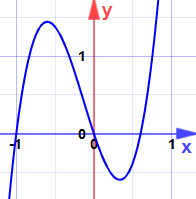## 再举个例

### 例子：求以下的极大值和极小值：

y = x3 − 6x2 + 12x − 5y = 3x2 − 12x + 12

y'' = 6(2) − 12 = 0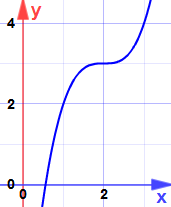## 一定要是可微分的。

### 例子：函数 f(x) = |x| （绝对值）

 |x| 的图像这样：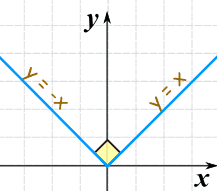（函数也一定要是 连续的，但任何可微分的函数都是连续的。）